#### Chapter 1 Number System R.D. Sharma Solutions for Class 9th Math Exercise 1.2

Exercise 1.2

1. Express the following rational numbers as decimals:
(i) 42/100
(ii) 327/500
(iii) 15/4

Solution

(i) Given rational number is 42/100
Now we have to express this rational number into decimal form. So we will use long division method as below.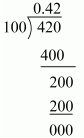Hence, 42/100 = 0.42

(ii) Given rational number is 327/500
Now we have to express this rational number into decimal form. So we will use long division method as below.Hence,  327/100 = 0.654

(iii) Given rational number is 15/4
Now we have to express this rational number into decimal form. So we will use long division method as below.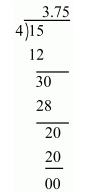Hence, 15/4 = 3.75

2. Express the following rational numbers as decimals:
(i) 2/3
(ii) -4/9
(iii) -2/15
(iv) -22/13
(v) 437/999
(vi) 33/26

Solution

(i) Given rational number is 2/3
Now we have to express this rational number into decimal form. So we will use long division method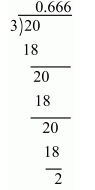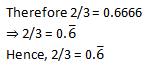(ii) Given rational number is -4/9
Now we have to express this rational number into decimal form. So we will use long division method

(iii) Given rational number is -2/15
Now we have to express this rational number into decimal form. So we will use long division method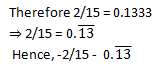(iv) Given rational number is -22/13
Now we have to express this rational number into decimal form. So we will use long division method(v) Given rational number is 437/999
Now we have to express this rational number into decimal form. So we will use long division method3. Look at several examples of rational numbers in the form p/q (q ≠0),p/q (q ≠0), where p and q are integers with no common factors other than 1 and having terminating decimal representations. Can you guess what property q must satisfy ?

Solution

Prime factorization is the process of finding which prime numbers you need to multiply together to get a certain number. So prime factorization of denominators (q) must have only the power of 2 or 5 or both.